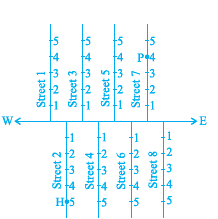# NCERT Class 9 Maths Coordinate Geometry

Read and download NCERT Class 9 Maths Coordinate Geometry chapter in NCERT book for Class 9 Mathematics. You can download latest NCERT eBooks chapter wise in PDF format free from Studiestoday.com. This Mathematics textbook for Class 9 is designed by NCERT and is very useful for students. Please also refer to the NCERT solutions for Class 9 Mathematics to understand the answers of the exercise questions given at the end of this chapter

## NCERT Book for Class 9 Mathematics Chapter 3 Coordinate Geometry

Class 9 Mathematics students should refer to the following NCERT Book chapter Chapter 3 Coordinate Geometry in standard 9. This NCERT Book for Grade 9 Mathematics will be very useful for exams and help you to score good marks

### Chapter 3 Coordinate Geometry NCERT Book Class 9

3.1 Introduction You have already studied how to locate a point on a number line. You also know how to describe the position of a point on the line. There are many other situations, in which to find a point we are required to describe its position with reference to more than one line. For example, consider the following situations: I. In Fig. 3.1, there is a main road running in the East-West direction and streets with numbering from West to East. Also, on each street, house numbers are marked. To look for a friend’s house here, is it enough to know only one reference point? For instance, if we only know that she lives on Street 2, will we be able to find her house easily? Not as easily as when we know two pieces of information about it, namely, the number of the street on which it is situated, and the house number. If we want to reach the house which is situated in the 2nd street and has the number 5, first of all we would identify the 2nd street and then the house numbered 5 on it. In Fig. 3.1, H shows the location of the house. Similarly, P shows the location of the house corresponding to Street number 7 and House number 4.Please refer to attached file for NCERT Class 9 Maths Coordinate Geometry

## Books recommended by teachers

 NCERT Class 9 Maths Number Systems NCERT Class 9 Maths Number Systems Questions
 NCERT Class 9 Maths Polynomials NCERT Class 9 Maths Polynomials Questions
 NCERT Class 9 Maths Coordinate Geometry NCERT Class 9 Maths Coordinate Geometry Questions
 NCERT Class 9 Maths Linear Equations In Two Variables NCERT Class 9 Maths Linear Equations In Two Variables Questions
 NCERT Class 9 Maths Introduction To Euclids Geometry NCERT Class 9 Maths Introduction To Euclids Geometry Questions
 NCERT Class 9 Maths Lines And Angles NCERT Class 9 Maths Lines And Angles Questions
 NCERT Class 9 Maths Triangles NCERT Class 9 Maths Triangles Questions